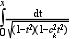Scilab Home page | Wiki | Bug tracker | Forge | Mailing list archives | ATOMS | File exchange
Change language to: Français - Português - 日本語

See the recommended documentation of this function

Scilab help >> Elementary Functions > delip

# delip

complete and incomplete elliptic integral of first kind

### Calling Sequence

`[r]=delip(x,ck)`

### Arguments

x

real vector with non negative elements

ck

real number between -1 and 1

r

real or complex number (or vector) with the same size as `x`

### Description

The elliptic integral of the first kind with parameter `ck` is defined as follow:Where `x` is real and positive, `ck` is in `[-1 1]`.

If x is less than 1 the result is real.

When called with `x` a vector `r` is evaluated for each entry of `x`.

### Examples

```ck=0.5;
delip([1,2],ck)
deff('y=f(t)','y=1/sqrt((1-t^2)*(1-ck^2*t^2))')
intg(0,1,f)    //OK since real solution!```

• amell — Jacobi's am function
• %asn — elliptic integral
• %sn — Jacobi 's elliptic function